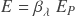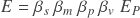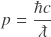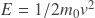# Energy

The New Foundation Model gives a single formula describing the energy of particles without rest mass and the kinetic energy of particles with rest mass. The essence of this formula is the 2-part energy mechanism describing a particle’s wavelength and velocity. The mechanism multiplies velocity by the strength of a particle’s wavelength, quantified naturally in unit dimension M but historically in unit dimensions LMT-1. Applying a spin operator gives the correct quantity of energy for any particle or system.

For particles without rest mass, the velocity and spin operators are both equal to 1. Consequently, there is no reduction in potential due to spin or velocity, and energy is determined entirely by wavelength. This behavior is evident in Photons.

Field resistance on particles with rest mass reduces their kinetic energy potential. A particle’s wavelength and inertial mass are thus limited to the scale of its shortest wavelength in non-relativistic terms. Both parts of the 2-part energy mechanism reduce the particle’s kinetic energy, increasing the wavelength and reducing the velocity equally. Kinetic energy is also reduced by a factor of one-half due to an extended cycle of half-spin particles.

## Standard formula

The standard energy formula applies the spin, wavelength, and velocity operators to the Planck energy potentialThe five unit dimensions of energy quantify the particle’s spin, wavelength, and velocity as shown in the particle mechanics template:

Applying the three operators to the maximum potential in each unit dimension produces observable quantities of wavelength, inertial mass, and velocity. The following illustration applies the operators to particles with no rest mass

### Observable

Particles with rest mass use the following forms of the operators

### Observable

The standard form of the energy formula can be stated using operators in each of the unit dimensions multiplied by the Planck energy. The standard formula produces the following for particles with rest mass

The standard form of the energy formula also works for particles without rest mass but uses the right values of spin and velocity for these particles. The spin and velocity operators do not affect the energy but the operators are included to show how the formula’s structure is the same for particles with and without rest mass.

The standard energy formula can be abbreviated for particles with no rest mass, showing only the wavelength operator which reduces the Planck energy potentialThis simple formula has three forms in the fundamental unit dimensions

## Expanded formula

The energy formula also uses the expanded form of the wavelength operator to find the energy. Replacing the wavelength operator with the equivalent rest mass and momentum operators gives the following expanded form of the energy formulaThe expanded formula produces the same observables from a different but equivalent set of operators

### Observable

The expanded form of the energy formula produces the following forms using unit dimensions of length, mass, and time in the operators

The simplest form of the energy formula uses the time dimension to summarize all of the operators. The formula stated for particles with and without rest mass is

#### rest mass

This formula demonstrates how elementary particles may be characterized as spatial and temporal distributions of a conserved Planck energy potential. A particle’s energy is determined simply by its oscillation period with the relevant spin factor applied.

## Observable formulas

The energy formula can be expressed using a particle’s observable properties of wavelength, inertial mass, and oscillation period. In traditional units of energy–which contain the unnecessary unit dimensions of length and time–these observables must be multiplied by the speed of light

#### rest mass

The formula for particles with no rest mass is equal to the historical formula ℏ/𝑇, and is half that amount for particles with rest mass.

The most natural representation of a particle’s kinetic energy is in units dimensions LMT-1. Removing 𝑐 from the formulas produces the kinetic energy in these dimensions

## Historical energy formulas

The New Foundation Model energy formula works for all types of elementary particles and systems. Different formulas have been used historically to calculate the energy of particles with rest mass versus those without. The formula for calculating a photon’s energycan be converted to the New Foundation Model energy formula by replacing Planck’s constant with fundamental units of length, mass, and time as shown in the following illustration

Photon energy: conversion from historical formula to natural formula

The classical kinetic energy formulacan also be converted to the New Foundation Model formula by showing the physical attributes of wavelength in place of the mathematically equivalent rest mass and velocity terms as shown in the following illustration

Kinetic energy: conversion from historical formula to natural formula